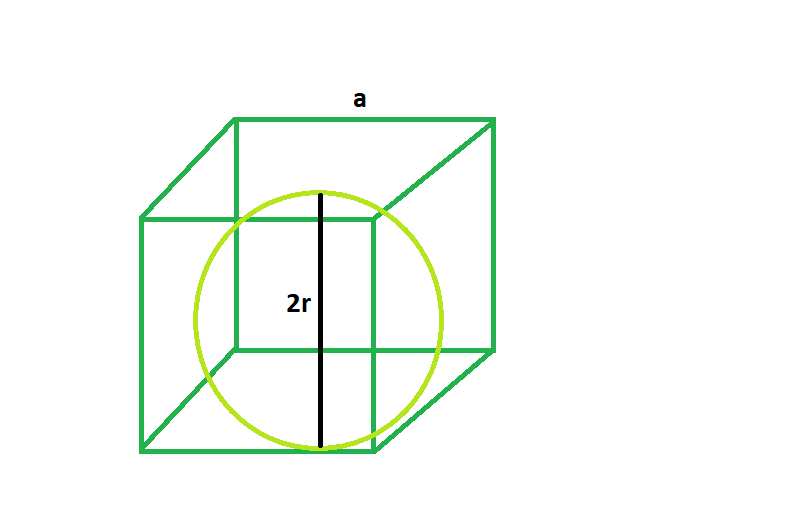# Largest sphere that can be inscribed inside a cube

Given here is a cube of side length a, the task is to find the biggest sphere that can be inscribed within it.

Examples:

```Input: a = 4
Output: 2

Input: a = 5
Output: 2.5
```Approach:

From the 2d diagram it is clear that, 2r = a,
where, a = side of the cube
r = radius of the sphere
so r = a/2.

Below is the implementation of the above approach:

## C++

 `// C++ Program to find the biggest sphere ` `// inscribed within a cube ` `#include ` `using` `namespace` `std; ` ` `  `// Function to find the radius of the sphere ` `float` `sphere(``float` `a) ` `{ ` ` `  `    ``// side cannot be negative ` `    ``if` `(a < 0) ` `        ``return` `-1; ` ` `  `    ``// radius of the sphere ` `    ``float` `r = a / 2; ` ` `  `    ``return` `r; ` `} ` ` `  `// Driver code ` `int` `main() ` `{ ` `    ``float` `a = 5; ` `    ``cout << sphere(a) << endl; ` ` `  `    ``return` `0; ` `} `

## Java

 `// Java Program to find the biggest sphere ` `// inscribed within a cube ` ` `  `class` `GFG{ ` `// Function to find the radius of the sphere ` `static` `float` `sphere(``float` `a) ` `{ ` ` `  `    ``// side cannot be negative ` `    ``if` `(a < ``0``) ` `        ``return` `-``1``; ` ` `  `    ``// radius of the sphere ` `    ``float` `r = a / ``2``; ` ` `  `    ``return` `r; ` `} ` ` `  `// Driver code ` `public` `static` `void` `main(String[] args) ` `{ ` `    ``float` `a = ``5``; ` `    ``System.out.println(sphere(a)); ` ` `  `} ` `} ` `// This code is contributed by mits `

## Python3

 `# Python 3 Program to find the biggest  ` `# sphere inscribed within a cube ` ` `  `# Function to find the radius  ` `# of the sphere ` `def` `sphere(a): ` `     `  `    ``# side cannot be negative ` `    ``if` `(a < ``0``): ` `        ``return` `-``1` ` `  `    ``# radius of the sphere ` `    ``r ``=` `a ``/` `2` ` `  `    ``return` `r ` ` `  `# Driver code ` `if` `__name__ ``=``=` `'__main__'``: ` `    ``a ``=` `5` `    ``print``(sphere(a)) ` ` `  `# This code is contributed  ` `# by SURENDRA_GANGWAR `

## C#

 `// C# Program to find the biggest  ` `// sphere inscribed within a cube  ` `using` `System; ` ` `  `class` `GFG ` `{ ` `// Function to find the radius  ` `// of the sphere  ` `static` `float` `sphere(``float` `a)  ` `{  ` ` `  `    ``// side cannot be negative  ` `    ``if` `(a < 0)  ` `        ``return` `-1;  ` ` `  `    ``// radius of the sphere  ` `    ``float` `r = a / 2;  ` ` `  `    ``return` `r;  ` `}  ` ` `  `// Driver code  ` `static` `public` `void` `Main () ` `{ ` `    ``float` `a = 5;  ` `    ``Console.WriteLine(sphere(a));  ` `}  ` `}  ` ` `  `// This code is contributed by ajit  `

## PHP

 ` `

Output:

```2.5
```

Don’t stop now and take your learning to the next level. Learn all the important concepts of Data Structures and Algorithms with the help of the most trusted course: DSA Self Paced. Become industry ready at a student-friendly price.

My Personal Notes arrow_drop_upCheck out this Author's contributed articles.

If you like GeeksforGeeks and would like to contribute, you can also write an article using contribute.geeksforgeeks.org or mail your article to contribute@geeksforgeeks.org. See your article appearing on the GeeksforGeeks main page and help other Geeks.

Please Improve this article if you find anything incorrect by clicking on the "Improve Article" button below.

Article Tags :
Practice Tags :

Be the First to upvote.

Please write to us at contribute@geeksforgeeks.org to report any issue with the above content.